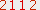﻿ Vector Inverter 002-Vector Inverters-Liyang Intelligent Energy Limited
Hotline:+86-020-81959520

Vector Inverter 002

The working principle of the inverter is to rectify and then inverter, after the inverter to get their own frequency voltage.
Online InquiryDetail

Vector frequency converter technology is based on the DQ axis theory, and its basic idea is to break the motor current D-axis current and Q-axis current, where the D-axis current is the excitation current, Q-axis current is the torque current, The AC motor has the same control characteristics as the DC motor. It is an ideal control theory designed for the AC motor, which greatly improves the control characteristic of the AC motor. However, the current control theory has not only applied to the AC asynchronous motor, DC inverter motor (BLDC, that is, permanent magnet synchronous motor) also extensive use of the control theory.
Vector and vector is a mathematical vector (vector) analysis of a method or concept, the two are the same concept, but the name is different, a simple definition is both the size and direction of the amount.
Vector is our (mainland) argument, the vector is generally used in Hong Kong and Taiwan literature. Meaning and "Bush" and "Bush" meaning roughly the same. Vector control is mainly a motor model decoupling concept.
In the field of electrical energy is mainly used for the analysis of AC power, such as motor analysis, the application in the inverter is based on the theory of motor analysis frequency control, called vector control inverter, the method is not unique, but the mathematical model Basically the same.